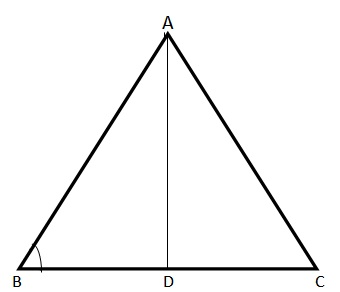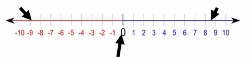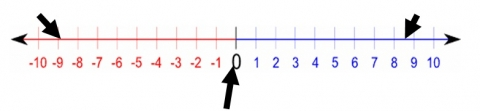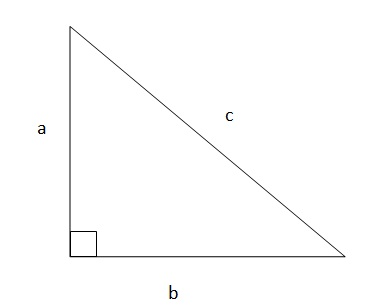# GoGMAT

 Categories : Triangles A triangle is a closed figure formed by three straight lines provided the sum of angles formed between the lines is 180 degrees. There are six types of triangles • Isosceles Triangle: A triangle with two sides of equal length and corresponding angles of equal measure. Example: Triangle with the angles 30°, 30° and 120°. • Equilateral Triangle: A triangle that has all three sides of equal length and all three angles of equal measure. As the sum of the three angles must equal 180°, each angle equals 60° in an equilateral triangle. • Scalene Triangle: If all the three sides of the triangle are of different size, then it is a scalene triangle. Example: A triangle with sides 3cm, 4cm and 5cm is scalene. • Right Triangle: A triangle that has one 90° angle. •    Acute Triangle: A triangle with all angles less than 90°.    Example: A triangle formed with angles 50°, 60° and 70° is an acute triangle • Obtuse Triangle: An obtuse triangle is a triangle with one angle greater than 90° o   Example: Triangle with angles 30°, 30° and 120°. Commonly used TermsAltitude: The perpendicular distance from the base to the opposite vertex. Base: We can take any side of the triangle as the base, for the purposes of further calculation.Vertex: Angles of triangles are called vertices. Any one of AB, BC or AC could be taken as the base. In this case, AD is the Altitude, and BC is the base. Angle A, Angle B and Angle C... Categories : Triangles Among all of the concepts explored in GMAT, triangles are a commonly revisited concept. When exploring triangles on the coordinate plane, it is important to understand the following terminology:• Ordered Pair: identification of a point through its coordinates, and is typically written in the form of (a, b) where a is a point on the x-axis, and b is a point on the y-axis • Origin: point of intersection of x-axis and y-axis, (0, 0) • Quadrants: a sector on the graph; there are 4 quadrants with coordinates of (+, +) for quadrant I, (-, +) for quadrant II, (-, -) for quadrant III, and (+, -) for quadrant IV • Slope: steepness of a line defined by the rise over run which is the units that are present vertically divided by the units present horizontallyUpon understanding the terminology, it is crucial to understand the basic equations regarding the distance between two points, or a line, in order to compose a more complex view on triangles.The basic equation will be y = mx + b where y is a point on the y-axis, m is the slope, x is a point on the x-axis, and b is the intercept on the y-axis.Since the GMAT normally provides a coordinate graph, counting the units on the y-axis, and the units on the x-axis will be relatively easy.For example, if two points on the graph have coordinates (0, 0) and (3, 6), we can find the slope by plugging the coordinates into the theorem of rise over run. The difference between the two points will be a value of 3 for the x-axis, and a value of 6 for the y-axis. The slope will be 6/3, which gives a value of 2. Finding the slope is crucial in determining many factors concerning a triangle, and will be often used to determine the coordinates of the three points.The most basic equation regarding calculating... Categories : Absolute Value, InequalitiesGood news, test takers! You can pass the GMAT! Most of the questions are not that difficult. The difficulty in the test is in taking a mid-level question, (5x2 = 10) and rephrasing it in a more difficult way. (5 men walk into a bar. The bartender gives each man a beer for each of their hands. How many beers does the bartender distribute all together?) There is one exception to the rule, though: Absolute value inequalities.It’s a little tough, but not impossible to figure out absolute value inequalities! We’ll start with absolute value expression’s basic definitions, mathematically and conceptually.  Then we’ll use that basic understanding to solve equations and inequalities including absolute value expressions. Finally, we’ll deal with a concept-based application of the absolute value inequalities on the GMAT. Let’s go! Absolute positive - Everybody knows that |9|=9 and|-9|=9.  What’s so special about those slanted lines? How and why does it neutralize a number?  Those bars are the measure of the distance of the number within the bars and zero. -9 and 9 are both 9 units away from 0. Picture it!Let’s look at it those two ways1) The number within the bars will definitely turn positive and 2) The distance from zero to the number is our basis for digging deeper into absolute value. This concept becomes difficult when a... Categories : Geometry Problems Let us try to tackle the problem of Special Right Triangles and Pythagorean Triplets, today. The theory will be followed by suitable examples for special right triangles. As you must be aware, the Pythagorean Theorem is one of the most used tools in geometry.  Its three main features are: 1) One of the angles is a right angle2) The sides which are adjacent to the right angle are termed as the base and perpendicular/height 3) The third side is known as the hypotenuseFor the figure given below, the hypotenuse is ‘c’, the base is ‘b’ and the perpendicular or height is ‘a’.  As proposed by Pythagoras, the sum of square of sides adjacent to right angle is equal to the square of the third side, better known as the hypotenuse. Putting these words into equation form, we see:                      a^2+ b^2=c^2Two major takeaways from this theorem are:a) It is applicable only to right angled trianglesb) The choice of base and perpendicular is irrelevant as far as the two sides adjacent to the right angled triangle are named.Let us quickly see one application of Pythagorean Theorem before moving on to Special Right Triangles. Example: John wants to visit his friend Ron’s house. He starts from his house and travels 3 km south before reaching a traffic signal. From there, he takes a 90 degree turn left and travels 4 km to reach Ron’s house. So how far is Ron’s house from John’s house? Answer:Let’s approach this question step by step... Categories : Absolute Value The GMAT focuses on measuring the candidate’s ability to solve quantitative problems and interpret data. The concept of absolute value inequalities have been known to be the most challenging. With the GMAT being a tightly timed examination, it is crucial that candidates dissect the questions quickly and come up with the correct answers. Let us look at the secrets of mastering absolute value inequalities.An inequality is a comparison between two values or expression where either values or expressions are not equal to one another. Most of the algebraic manipulations that are used in mathematical expressions can be applied to inequalities; however, there are several exceptions listed below that need to be applied. These points may seem trivial; however, the consequences can be severe, and can mislead candidates to the wrong answers.1) When an inequality is being multiplied by a negative value, the direction of the sign must be switched. 2) Unlike expressions, inequalities cannot be squared. This means that a > y will not equal to a^2 > y^2. 3) The square root of both sides cannot be taken to obtain an answer. Inequalities with an absolute value term will typically have two solutions. In order to simplify solving absolute value inequalities on the GMAT examination, candidates should follow these steps: 1)    Isolate the absolute value expression found in the inequality 2)    Set the expression so that it equals to both a positive and a negative value and 3)    Solve for the inequality. Let’s take a look an inequality with only one absolute value term.        5 +  | 2x + 1 | < 10Let us follow the shortcuts1) First, we will isolate the absolute term from the inequality, so that...

Get F1GMAT's Newsletters (Best in the Industry)
Included in the Newsletter:

• Ranking Analysis
• Post-MBA Salary Trends
• Post-MBA Job Function & Industry Analysis
• Post-MBA City Review
• MBA Application Essay Tips
• School Specific Essay Tips
• GMAT Preparation Tips
• MBA Admission Interview Tips
• School Specific Interview Tips
• Funding Guidance and
• Special Consultation Service (only for Subscribers)

Subscribe to F1GMAT's Newsletter